# thetaticks

Set or query theta-axis tick values

## Syntax

``thetaticks(ticks)``
``tv = thetaticks``
``thetaticks('auto')``
``thetaticks('manual')``
``m = thetaticks('mode')``
``___ = thetaticks(pax,___)``

## Description

example

````thetaticks(ticks)` sets the theta-axis tick values, which are the locations along the theta-axis where the tick marks and grid lines appear. Specify `ticks` as a vector of increasing values; for example, `[0 90 180 270]`. This command affects the current axes.```
````tv = thetaticks` returns the current theta-axis tick values as a vector.```

example

````thetaticks('auto')` sets an automatic mode, enabling the axes to determine the theta-axis tick values. Use this option if you change the tick values and then want to set them back to the default values. ```
````thetaticks('manual')` sets a manual mode, freezing the theta-axis tick values at the current values. Use this option if you want to retain the current tick values when resizing the axes or adding new data to the axes.```
````m = thetaticks('mode')` returns the current theta-axis tick labels mode, which is either `'auto'` or `'manual'`. By default, the mode is automatic unless you specify tick values or change the mode to manual.```

example

````___ = thetaticks(pax,___)` uses the axes specified by `pax` instead of the current axes. Specify `pax` as the first input argument for any of the previous syntaxes.```

## Examples

collapse all

Create a polar plot. Display tick marks and grid lines along the theta-axis at 0, 90, 180, and 270 degrees. Then, specify a label for each tick mark.

```theta = linspace(0,2*pi); rho = theta/10; polarplot(theta,rho) thetaticks([0 90 180 270]) thetaticklabels({'East','North','West','South'})```Display tick marks along the theta-axis at nonuniform values between 0 and 360 degrees.

```theta = linspace(0,2*pi); rho = theta/10; polarplot(theta,rho) thetaticks([0 30 60 90 180 210 240 270])```Display tick marks along the theta-axis every 15 degrees.

```theta = 0:0.01:2*pi; rho = sin(2*theta).*cos(2*theta); polarplot(theta,rho) thetaticks(0:15:360)```Create a polar plot and specify the theta-axis tick values. Then, set the theta-axis tick values back to the default values.

```polarplot(1:10) thetaticks([0 120 240])```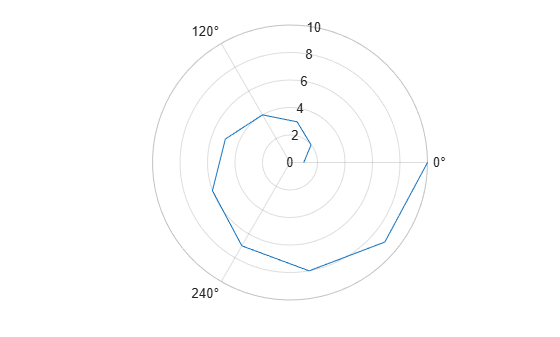`thetaticks('auto')`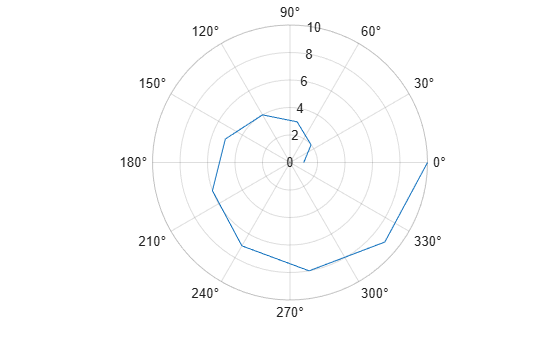Create polar axes and return the polar axes object `pax`. Ensure that `thetaticks` affects the polar axes you just created by passing `pax` as the first input argument to the function.

```pax = polaraxes; thetaticks(pax,0:45:360)```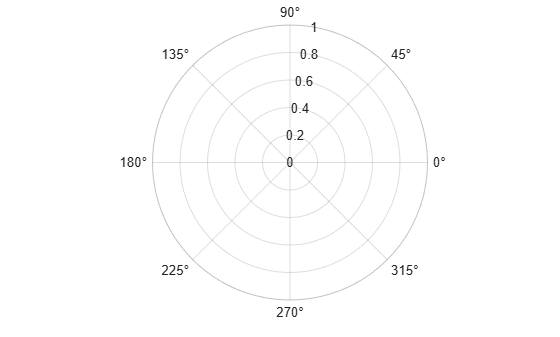Remove the tick marks and grid lines along the theta-axis by specifying the tick values as an empty array.

```theta = 0:0.01:2*pi; rho = sin(2*theta).*cos(2*theta); polarplot(theta,rho) thetaticks([])```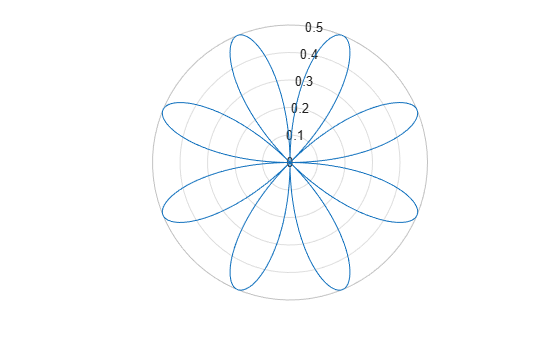## Input Arguments

collapse all

Tick values, specified as a vector of increasing values. The tick values are interpreted in units determined by the `ThetaAxisUnits` property. By default, the units are degrees.

If you do not want tick marks along the theta-axis, specify an empty vector `[]`.

Example: `thetaticks([0 90 180 270])`

Example: `thetaticks([0:30:330])`

Example: `thetaticks([])`

### Note

To specify the tick labels, use the `thetaticklabels` function.

Polar axes, or an array of polar axes. If you do not specify this argument, then `thetaticks` modifies the current axes (provided that the current axes is a polar axes object).

## Output Arguments

collapse all

Current tick values, returned as a vector.

Current mode, returned as one of these values:

• `'auto'` — Axes automatically determines the theta-axis tick values.

• `'manual'` — Axes uses manually specified theta-axis tick values.

collapse all

### Tick Values

The tick values are the locations along the theta-axis where the tick marks appear. The tick labels are the labels that you see next to each tick mark. Set the tick values using the `thetaticks` function. Set the corresponding tick labels using the `thetaticklabels` function.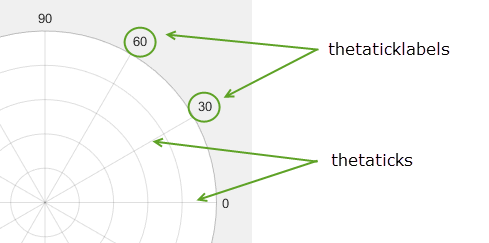## Algorithms

The `thetaticks` function sets and queries several axes properties related to the theta-axis tick values.

• `ThetaTick` — Property that stores the theta-axis tick values.

• `ThetaTickMode` — Property that stores the theta-axis tick value mode. When you set the theta-axis tick values, this property changes to `'manual'`.